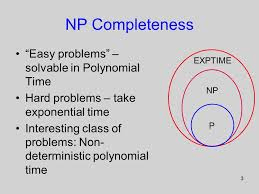# Stop Looking For the “Optimal” Production Scheduling Solution

Which of you readers like computational math? If you do, jump to the next paragraph, if you don’t I’ll cut to the chase – understanding that finding a mathematical solution that produces an “optimal” production schedule is a more challenging version of the Travelling Salesman problem, our dilemma of finding a solution then falls into the class of “Non-deterministic Polynomial” problems and therefore, it is unsolvable. There is actually a Wikipedia entry on it if you’re really into this stuff.For those of you who want a little more, read on. As computing power has increased, those in academia continue to think about what types of problems could be solved, and perhaps as importantly, what types of problems could NOT be solved with computers (thus was born the field of Computational Complexity within computer science and mathematics). This field of study essentially boils down to providing a method of determining whether a particular problem can be solved using a computer in a reasonable amount of time.

#### Some Problems Can Be Solved in A Reasonable Amount of Time (“Polynomial”)

Some problems take a reasonable amount of time and can easily be expected to be completed by a computer within microseconds, or worst case, hours or days, such as sorting N number of inputs (say, names and addresses records, for example). Or finding duplicates in N number of records. These types of problems are classified as being able to be solved by a computer within a “polynomial” amount of time. In other words, there exists a polynomial type expression (or smaller in magnitude) that describes the time complexity of the algorithm to solve the problem. Like N2 +8N + 9. In the field of computational complexity, these problems are classified as belonging to the set of “P” problems for “polynomial”.#### Some Cannot (“Non-deterministic Polynomial”)

There are then another set or class of problems that cannot be solved in polynomial time, and at minimum take an exponential amount of time based on the size of the number of inputs. For example, at best, this sort of problem can be solved in 2N time.

To get a direct experience of the magnitude of the difference, take a problem of say 80 input values, and an algorithm that is in the class of “P” or polynomial type problems, would be able to be solved in say,

802 +8*80 + 9 = 7,049, say nanoseconds, so very quick, not even a second and you know the answer.

However, take a problem that can be solved minimally in 2N time, with the same number of input values at 80:

280 = 1,208,925,819,615,000,000,000,000 time units. If time units = nanoseconds, that would be about 38 Million years.

So, any such problem is referred to as “Non-deterministic Polynomial” or simply, in the set NP class of problems.

#### The Traveling Salesperson problem

The Traveling Salesperson problem is one such problem. Take N number of cities, and the distances between each pair of cities, solve for the overall shortest route hitting each city once and ending in the city you started. The time complexity to solve this problem is actually quite a bit worse than exponential (2N), it’s factorial in nature or N! (1x2x3x4x5x6x….xN) which gets crazy big crazy fast – try it on a calculator: N! passes the value of 280 at only N=25.

There is actually a movie called “Travelling Salesman” that came out in 2012 that explores the idea, “What if someone found a method to eliminate P vs NP?”, in other words, what if you could actually solve any NP class problem in polynomial time? The fact that all the world’s governments use cyber security methods can only be solved by NP type algorithms, makes this an very interesting proposition and a high drama type of movie as whichever country’s government had this information would have a significant upper hand in being able to override any of their secret-coded security protocols.

#### The Unicorn Known As The “Optimal” Production Schedule

Understanding that finding a mathematical solution that produces an “optimal” production schedule is a more challenging version of the Travelling Salesman problem, our dilemma of finding a solution then falls into the class of “Non-deterministic Polynomial” problems and therefore, it is unsolvable. Again, is actually a Wikipedia entry on it if you’re really into this stuff.

There is no such thing as an “Optimal” production schedule. So what exactly are we expecting our ERP and APS systems to be doing for us? Click here for our blog discussing the 3 Reasons Why Production Scheduling is Not Working for You.

#### See How PFM Can Benefit Your Shop

Drastically improve on-time delivery by prioritizing what needs to happen first, not what’s due first.